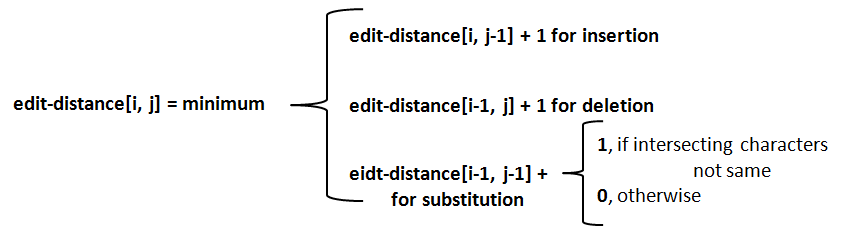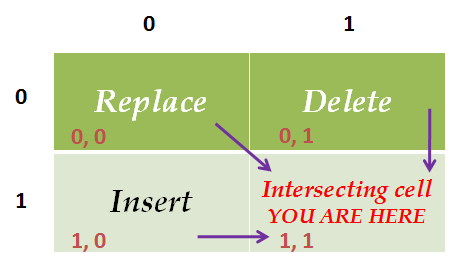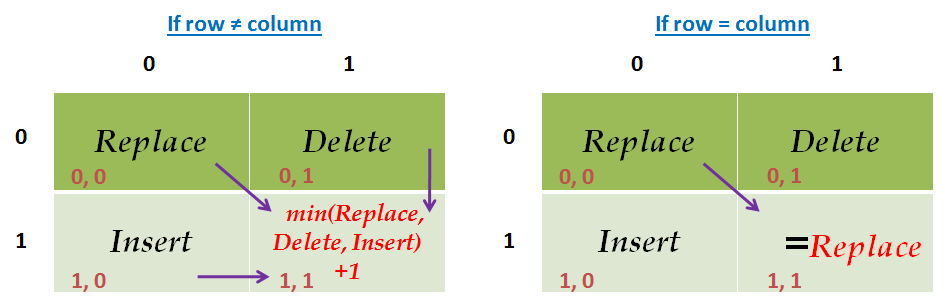Advanced Database Management System - Tutorials and Notes: Find minimum edit distance between strings

## Find minimum edit distance between two strings

Question:
Find the minimum edit distance in transforming the word DOG to COW using insertion, deletion, and substitution cost as 1.

Solution:
Minimum edit distance is the minimum number of editing operations (insertion, deletion, substitution) required to convert one string into another. The solution given here uses Basic Minimum Edit Distance which uses cost for all operations as 1.
Each cell in the distance matrix contains the distance between two strings. For instance, the cell intersect at i, j (distance[i, j]) contains the distance between first i characters of the target and the first j characters of the source. The value for each cell is calculated as per the equation shown below;Step 1: Draw the edit distance matrix. In this, each word is preceded by # symbol which marks the empty string.
 # C O W # D O G

Step 2: Find the edit-distance values using minimum edit distance algorithm to convert # (row side) to #COW (column side) and populate appropriate cells with the calculated distance.
Number of operations required to convert;
• # to # is 0.
• # to #C is 1. That is, one insertion (no change with #, insert C)
• # to #CO is 2. That is, two insertions (no change with #, insert C and O)
• # to #COW is 3. That is, three insertions (no change with #, insert C, O and W)
 # C O W # 0 1 2 3 D O G

Step 3: Find the edit-distance values using minimum edit distance algorithm to convert # (column side) to #DOG (row side) and populate appropriate cells with the calculated distance.
Number of operations required to convert
• # to # is 0.
• # to #D is 1. [one insertion (no change with #, insert D]
• # to #DO is 2. [two insertions (no change #, insert D and O]
• # to #DOG is 3. [three insertions (no change #, insert D, O and G]
 # C O W # 0 1 2 3 D 1 O 2 G 3

So far, we have found the minimum edit distance for 7 sub-problems.
[# - # = 0, # - #C = 1, # - #CO = 2, # - #COW = 3, # - #D = 1, # - #DO = 2, and # - #DOG = 3]

Step 4: From this step onward, we try to find the cost for a sub-problem by finding the minimum cost of three sub-problems and add 1 with that if the characters intersect at that cell are different. If the intersecting characters are same, then we add 0 with the diagonal cell value.
[Note: we have used A as the name for this matrix and included the index numbers for easy understanding]

From this step onward, use the following table as a key to find the cost.If the row character ≠ column character,
• The cost of the intersecting cell = min(replace, delete, insert) + 1.
If the row character = column character,
• The cost of the intersecting cell = cost of the Replace cell[Note: If row = column, then the cost of the intersecting cell is the cost of the sub-problem, ie., the cell at diagonal above]
Edit distance for Row 1

Sub-problem: #D #C.
Intersecting cell: A[1, 1]
Same intersecting characters? NO.
Cost(A[1, 1])       = Min(A[0, 0], A[0, 1], A[1, 0]) + 1
= A[0, 0] + 1
= 0 + 1 = 1
 A 0 1 2 3 # C O W 0 # 0 1 2 3 1 D 1 1 2 O 2 3 G 3

Sub-problem: #D #CO.
Intersecting cell: A[1, 2]
Same intersecting characters? NO.
Cost(A[1, 2])       = Min(A[0, 1], A[0, 2], A[1, 1]) + 1
= A[1, 1] + 1
= 1 + 1 = 2
 A 0 1 2 3 # C O W 0 # 0 1 2 3 1 D 1 1 2 2 O 2 3 G 3

Sub-problem: #D #COW.
Intersecting cell: A[1, 3]
Same intersecting characters? NO.
Cost(A[1, 3])       = Min(A[0, 2], A[0, 3], A[1, 2]) + 1
= A[1, 2] + 1
= 2 + 1 = 3
 A 0 1 2 3 # C O W 0 # 0 1 2 3 1 D 1 1 2 3 2 O 2 3 G 3
Edit distance for Row 2

Sub-problem: #DO #C.
Intersecting cell: A[2, 1]
Same intersecting characters? NO.
Cost(A[2, 1])       = Min(A[1, 0], A[1, 1], A[2, 0]) + 1
= A[1, 0] + 1
= 1 + 1 = 2
 A 0 1 2 3 # C O W 0 # 0 1 2 3 1 D 1 1 2 3 2 O 2 2 3 G 3

Sub-problem: #DO #CO.
Intersecting cell: A[2, 2]
Same intersecting characters? YES
Cost(A[2, 2])       = Min(A[1, 1], A[1, 2], A[2, 1]) + 0
= A[1, 1] + 0
= 1 + 0 = 1
 A 0 1 2 3 # C O W 0 # 0 1 2 3 1 D 1 1 2 3 2 O 2 2 1 3 G 3

Sub-problem: #DO #COW.
Intersecting cell: A[2, 3]
Same intersecting characters? NO.
Cost(A[2, 3])       = Min(A[1, 2], A[1, 3], A[2, 2]) + 1
= A[2, 2] + 1
= 1 + 1 = 2
 A 0 1 2 3 # C O W 0 # 0 1 2 3 1 D 1 1 2 3 2 O 2 2 1 2 3 G 3
Edit distance for Row 3

Sub-problem: #DOG #C.
Intersecting cell: A[3, 1]
Same intersecting characters? NO.
Cost(A[3, 1])       = Min(A[2, 0], A[2, 1], A[3, 0]) + 1
= A[2, 0] + 1
= 2 + 1 = 3
 A 0 1 2 3 # C O W 0 # 0 1 2 3 1 D 1 1 2 3 2 O 2 2 1 2 3 G 3 3

Sub-problem: #DOG #CO.
Intersecting cell: A[3, 2]
Same intersecting characters? NO
Cost(A[3, 2])       = Min(A[2, 1], A[2, 2], A[3, 1]) + 1
= A[2, 2] + 1
= 1 + 1 = 2
 A 0 1 2 3 # C O W 0 # 0 1 2 3 1 D 1 1 2 3 2 O 2 2 1 2 3 G 3 3 2

Sub-problem: #DOG #COW.
Intersecting cell: A[3, 3]
Same intersecting characters? NO.
Cost(A[3, 3])       = Min(A[2, 2], A[2, 3], A[3, 2]) + 1
= A[2, 2] + 1
= 1 + 1 = 2
 A 0 1 2 3 # C O W 0 # 0 1 2 3 1 D 1 1 2 3 2 O 2 2 1 2 3 G 3 3 2 2
The last cell (A[3, 3]) holds the minimum edit distance between the given strings DOG and COW.

Alignment:

The alignment between DOG and COW is as follows;

D                 O                 G

|                 |                 |

C                 O                 W

Substitute           No          Substitute

Cost = 1           change        Cost = 1

*************

Go to NLP Glossary